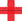top of page

## Video ResourcesIn this video, Luke or Lindsey takes students through a full Stats Medic EFFL lesson.

# Discrete Random Variables

##### Learning Targets
• Use the probability distribution of a discrete random variable to calculate the probability of an event.

• Make a histogram to display the probability distribution of a discrete random variable and describe its shape.

• Calculate and interpret the mean (expected value) of a discrete random variable.

bottom of page